# Ekeland variational principle

There are usually three ways for getting existence results in analysis, namely compactness, Hahn–Banach-type results and completeness properties (cf. Compactness; Hahn–Banach theorem; Completeness (in topology)). The Ekeland variational principle [a10] (which provides a characterization of complete metric spaces [a14], cf. also Complete metric space) illustrates the third method in the framework of optimization. Letbe a lower semi-continuous function defined on a complete metric space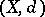, with values in the extended line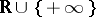, and bounded from below. It is well known that the lower bound ofoverneed not be attained. Ekeland's basic principle asserts that there exists a slight perturbation ofwhich attains its minimum on. More precisely, there exists a pointsuch that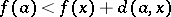for all; this says that the functionhas a strict minimum onat. It is interesting to observe that the conclusion of the basic principle is equivalent to the existence of a maximal element in the epigraph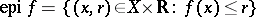for the order defined on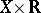by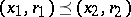if and only if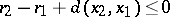[a3].Figure: e110030a

From this basic principle one can deduce some variants which are in fact equivalent to the basic statement. The first one is as follows: given,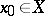such that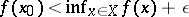and applying the basic principle to the complete metric space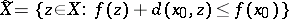, one obtains the existence of a pointsuch that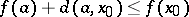andfor all. In particular, this implies that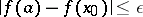. Applying the previous result with the metric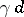,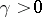, yields the second variant: there exists an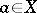such that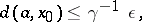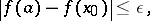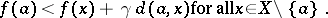This variational principle has several equivalent geometric formulations. For instance, the Phelps extremization principle and the Drop theorem [a7], [a12] (see [a13] for the versions as stated here). Letbe a closed subset of a Banach space, let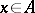and letbe a closed convex bounded subset ofsuch that. Then there exist a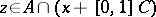and a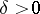such that.

Among the great number of applications is the celebrated Bröndsted–Rockafellar theorem in convex analysis [a6]. Letbe a closed convex function defined on a real Banach space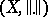with values in(cf. also Convex function (of a real variable)). Let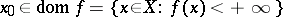,, and letbe such thatfor all. One can apply the third version of the theorem, with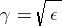, to the function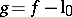when endowingwith the equivalent norm[a4]. This yields the existence of anand an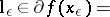such that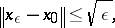Hence the set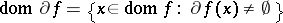is dense in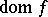for the epigraph topology, i.e. the supremum of the norm topology onand of the initial topology associated to.

Another easy consequence of the Ekeland variational principle is a generalization to multi-functions of the Kirk–Caristi fixed-point theorem [a2].

Finally, it should be mentioned that analogous results hold in Banach spaces with the perturbation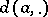replaced by some smooth one [a5].